# Finding equivalent fractions with visual fraction models

##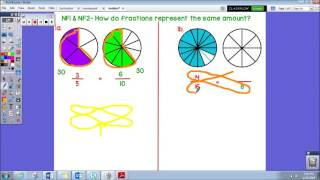By Amy Long

This video explains how to find an equivalent fraction using visual fraction models and also using cross multiplication.# Equivalent Fractions (3.NF.3B)

##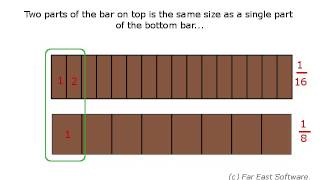By Far East Software

Recognize and generate simple equivalent fractions, e.g., 1/2 = 2/4, 4/6 = 2/3. Explain why the fractions are equivalent, e.g., by using a visual fraction model.# [3.NF.3b-1.4] Equivalent Fractions - Common Core Standard

##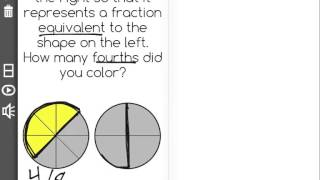By Freckle education

Students must explain why fractions are equivalent using a visual fraction model.# [4.NF.1-1.0] Generate Equivalent Fractions - Common Core Standard

##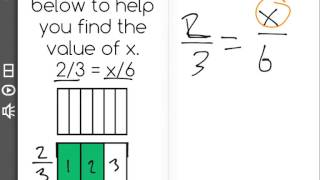By Freckle education

Explain why a fraction a/b is equivalent to a fraction (n x a) / (n x b) by using visual fraction models# Finding Equivalent Fractions using a Bar Model

##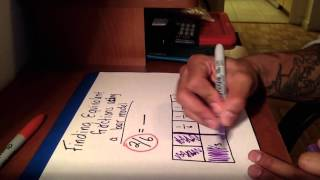By Math with Mr.OK

Explain equivalence of fractions in special cases, and compare fractions by reasoning about their size# Multiplying Fractions by Whole Numbers in Word Problems

##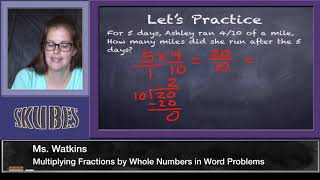By Skubes ed

Solve real world problems involving multiplication of fractions and mixed numbers, e.g., by using visual fraction models or equations to represent the problem# Using visuals to add and subtract fractions with unlike denominators

##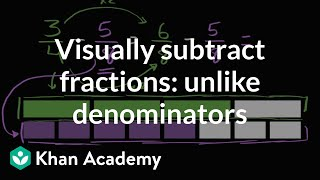By Khan Academy

Learn to subtract 3/4 - 5/8 using a fraction model for help.# Using visuals to add and subtract fractions with unlike denominators

##By Khan Academy

Learn to add 5/6 + 1/4 using a fraction model for help.# Writing Tenths and Hundredths with Decimals

##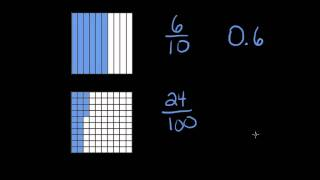By StoneBridgeMath

This video shows how pictures of tenths and hundreds are connected to both fractions and decimals. The instructor displays visual models then shades various areas in the models and she expresses the shaded areas as both a fraction and a decimal.# Equivalent fraction models

##By Khan Academy

Use same-sized wholes to show equivalent fractions.# Equivalent fractions on the number line

##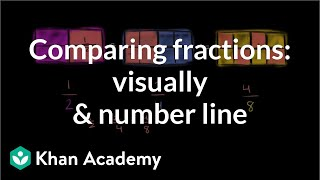By Khan Academy

Compare fractions by graphing them a number line and drawing fraction models.# Equivalent fractions on the number line

##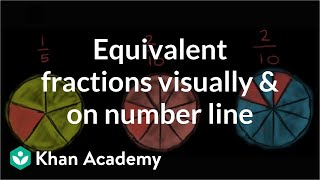By Khan Academy

Use number lines and fraction models to show equivalent fractions.# Equivalent fractions on the number line

##By Khan Academy

Create equivalent fractions by dividing a fraction model and number line into smaller parts.# Equivalent fractions on the number line

##By Khan Academy

Use number lines and fraction models to show equivalent fractions.# Equivalent fractions on the number line

##By Khan Academy

Compare fractions by graphing them a number line and drawing fraction models.# Finding 1 on the number line

##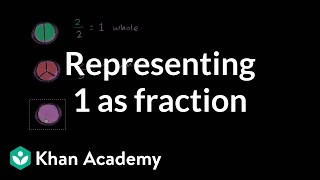By Khan Academy

Use fraction models and a number line to represent 1 as a fraction.# Equivalent fractions on the number line

##By Khan Academy

Compare fractions by graphing them a number line and drawing fraction models.# Finding 1 on the number line

##By Khan Academy

Use fraction models and a number line to represent 1 as a fraction.# Equivalent fractions on the number line

##By Khan Academy

Compare fractions by graphing them a number line and drawing fraction models.# Equivalent fractions on the number line

##By Khan Academy

Use number lines and fraction models to show equivalent fractions.